# How to use Foxta with CPT data?

July 20, 2018 by Terrasol

This document aims to show and clarify how to use Foxta with Cone Penetration Testing data (CPT) for shallow and deep foundations.

## Shallow foundations

Foxta is appropriate for the design of shallow foundations, including :

• isolated footings
• spread footings
• slab foundations...

Remember:

• Fondsup allows for the design of rectangular or circular isolated and spread footings.
• Tasplaq handles 3D modeling of pavements or rafts for any geometry and loading on a multilayer soil.
• Tasseldo provides estimations of settlements and stresses in a multilayer soil.

### Fondsup

Fondsup allows for the design of rectangular or circular isolated and spread footings based on CPT data.

#### Parameters tab

If CPT data are available, one has to define the design method as From penetrometer results.

In this case, we recommend to process by measurements.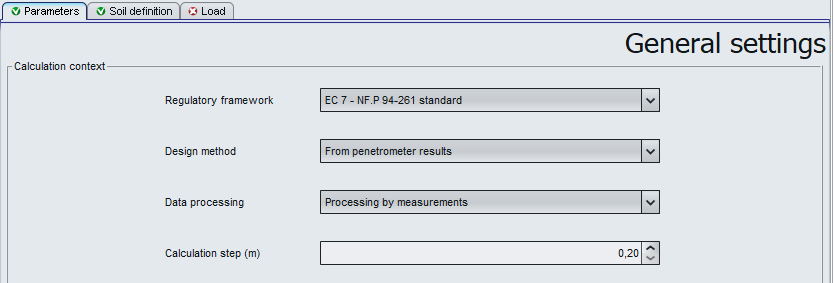#### Soil definition tab

• $q_c$ : tip resistance [kPa]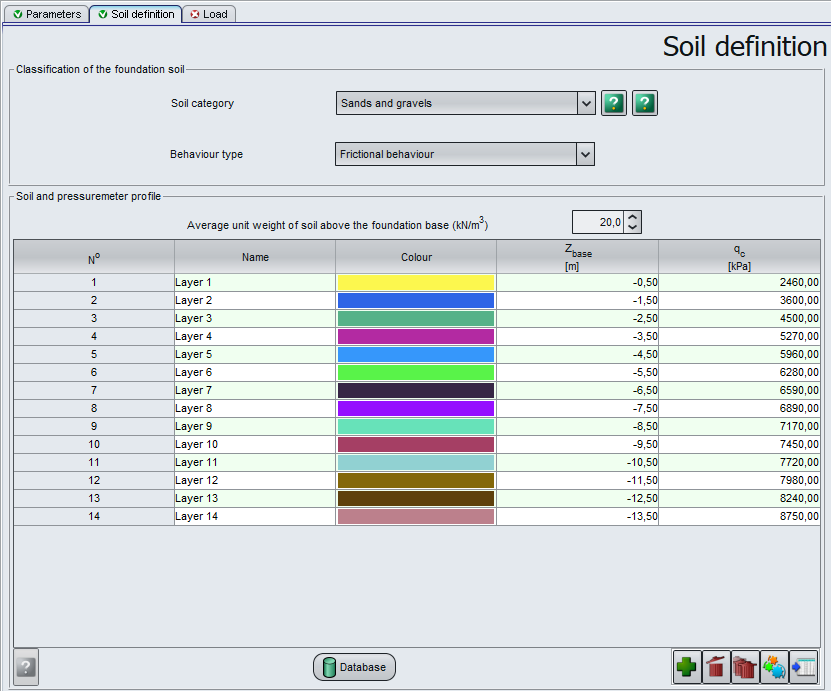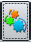The import wizard allows for the import a lot of data from clipboard (button Import).

For example, one may define all values in an Excel spreadsheet, select the range of data and then copy to clipboard.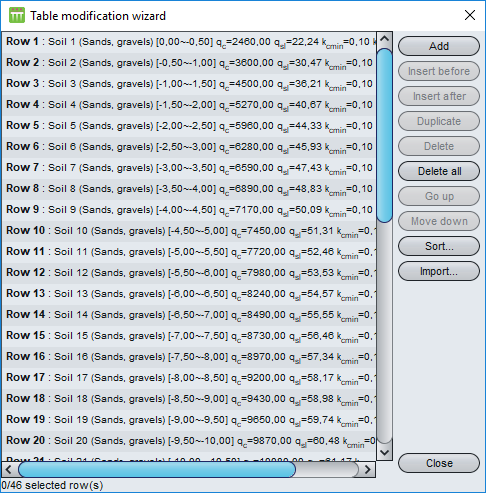### Tasplaq and Tasseldo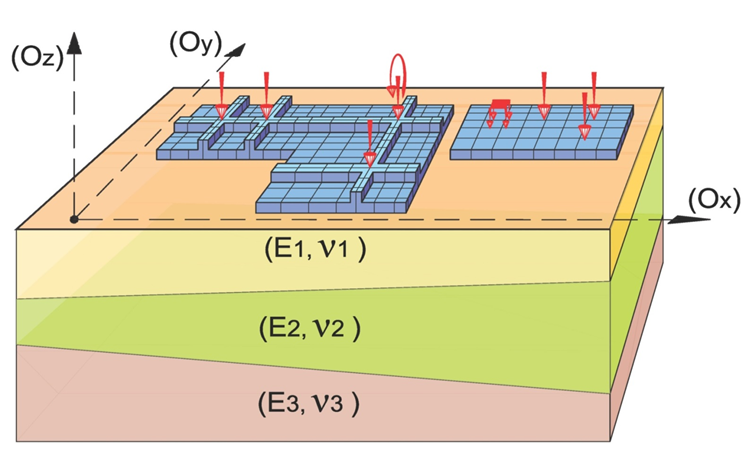Tasplaq model (Cuira & Simon 2006)

The Tasplaq and Tasseldo modules require the input of soil elastic modulus ($E_s$) values.

How to determine soil elastic modulus ($E_s$) from CPT data?

In case of an important level of shear strains (raft under localized load, shallow foundation, embankment or slope edge...), Eurocode 7 (NF P 94-261 J.2.2) suggest to use Robertson model:

• If $I_R<2.2$:

• $\alpha_E=0.015\cdot10^{0.55I_R+1.68}$
• If $I_R>2.2$:

• $\alpha_E=\frac{Q_T}2$for $Q_T<14$
• $\alpha_E=11.7$ for $Q_T>14$

In case of important volumetric strains ($\varepsilon_v$) (embankment on soft soil...), Eurocode 7 (NF P 94-261 J.2.3) suggest to use Sanglerat model:

## Deep foundations

Here are a few examples of deep foundations which may be designed with Foxta:

• piles (reinforced concrete, steel...)

• micropiles

• barrettes

• pile-raft foundations

• stiff inclusions...

Remember:

• Fondprof is used to evaluate the bearing capacity of a single deep foundation.

• Taspie+ handles :

• the calculation of pile and soil settlements in case of a single deep foundation under axial loading
• the design of stiff inclusions
• Piecoef+ provides fine calculations for single deep foundations under lateral loading.

• Groupie+ handles 3D calculations for a group of piles connected on top with an infinitely rigid footing.

### Fondprof

Fondprof is used to evaluate the bearing capacity of a single deep foundation.

#### Parameters tab

First of all, define the design method as From penetrometer results** in the Parameters tab.

After, you may chose to process by measurements or by layers. In the case of CPT data, it can be useful to process by measurements.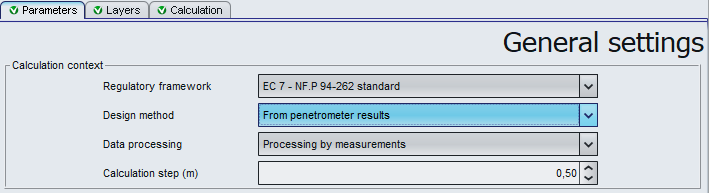#### Layers tab

We recommend to define one soil layer per $q_c$ value from CPT data.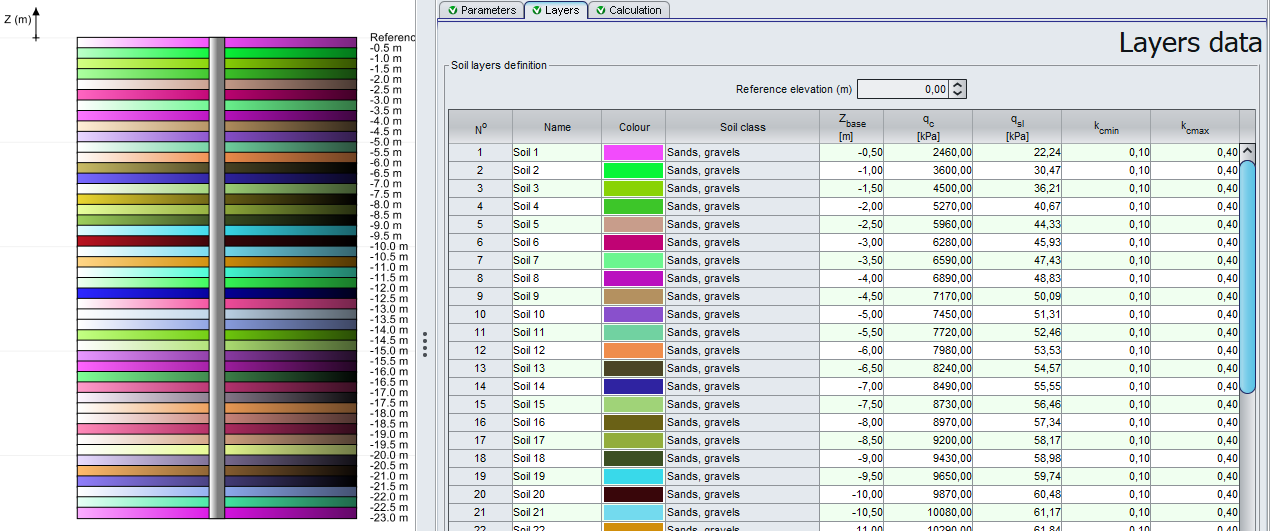##### Skin friction
• $q_c$ : tip resistance [kPa]the Import wizard allows for the import a large amount of data from clipboard (button Import).

So, one may define all values in an Excel spreadsheet, select a range and then copy to clipboard.

The Soil class values have to be imported by digits:

• 0 for No import
• 1 for Clays and silts
• 2 for Intermediate soils
• 3 for Sands and gravels
• 4 for Chalks
• 5 for Marls and marly limestones
• 6 for Weathered or fragmented rocks• $q_{sl}$ : skin friction [kPa]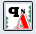a Wizard may be used to automatically calculate the $q_{sl}$ value for each input of $q_c$ .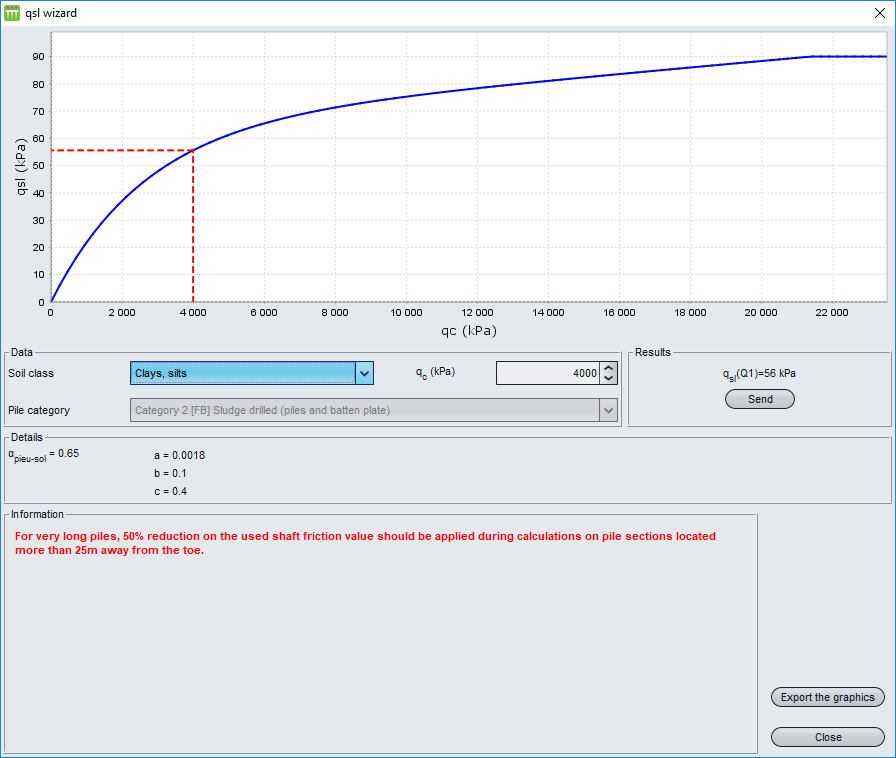$q_{sl}$ wizard available in Layers tab

##### Tip resistance

The $k_{c,min}$ and $k_{c,max}$ values may be defined by the user.

• $k_{c,min}$ : minimal penetrometric factor for bearing capacity calculation (no unit)

• $k_{c,min}=0.30$ for clay
• $k_{c,min}=0.20$ for intermediate soil
• $k_{c,min}=0.10$ for sands and graves
• $k_{c,min}=0.15$ for other cases (chalk, marls, altered or fragmented rock)
• $k_{c,max}$ : maximal penetrometric factor for bearing capacity calculation (no unit)

Final penetrometric factor for bearing capacity calculation will be obtained from the following expression: